# GATE Past Year Questions: Fluid Statics Notes | Study Fluid Mechanics - Mechanical Engineering

## Mechanical Engineering: GATE Past Year Questions: Fluid Statics Notes | Study Fluid Mechanics - Mechanical Engineering

The document GATE Past Year Questions: Fluid Statics Notes | Study Fluid Mechanics - Mechanical Engineering is a part of the Mechanical Engineering Course Fluid Mechanics.
All you need of Mechanical Engineering at this link: Mechanical Engineering

Try yourself:For the stability of a floating body the

[2017 : Set-2]

Try yourself:Consider a frictionless, massless and leak-proof plug blocking a rectangular hole of dimensions 2R × L at the bottom of an open tank as shown in the figure. The head of the plug has the shape of a semi-cylinder of radius R. The tank is filled with a liquid of density ρ up to the tip of the plug.
The gravitational acceleration is g. Neglect the effect of the atmospheric pressure.

[2016 : Set-1]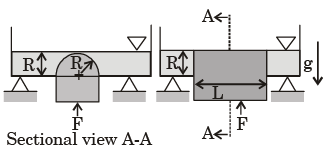The force F required to hold the plug in its position is

Try yourself:For a floating body, buoyant force acts at the

[2016 : Set-1]

*Answer can only contain numeric values
Try yourself:The large vessel shown in the figure contains oil and water. A body is submerged at the interface of oil and water such that 45 percent of its volume is in oil while the rest is in water.
The density of the body is _______ kg/m3.
The specific gravity of oil is 0.7 and density of water is 1000 kg/m3.
Acceleration due to gravity: g = 10 m/s2.

[2016 : Set-2]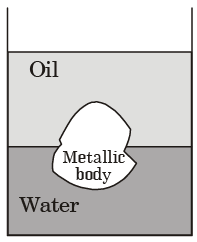Try yourself:For a completely submerged body with centre of gravity G and centre of buoyancy B, the condition of stability will be

[2014 : Set-1]

Try yourself:A hinged gate of length 5 m, inclined at 30° with the horizontal and with water mass on its left, is shown in figure below. Density of water is 1000 kg/m3. The minimum mass of the gate in kg per unit width (perpendicular to the plane of paper), required to keep it closed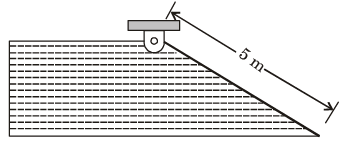Try yourself:A large tank with a nozzle attached contains three immiscible, inviscid fluids as shown. Assuming that the changes in h1, h2 and hare negligible, the instantaneous discharge velocity is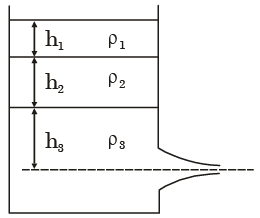Try yourself:For the stability of a floating body, under the influence of gravity alone, which of the following is TRUE?



Try yourself:Oil in a hydraulic cylinder is compressed from an initial volume 2m3 to 1.96 m3. If the pressure of oil in the cylinder changes from 40 MPa to 80 MPa during compression, the bulk modulus of elasticity of oil is



Try yourself:The pressure gauges G1 and G2 installed on the system show pressure of ρG1 = 5.00 bar and ρG2 = 1.00 bar. The value of unknown pressure P is [Atmospheric pressure = 1.01 bar]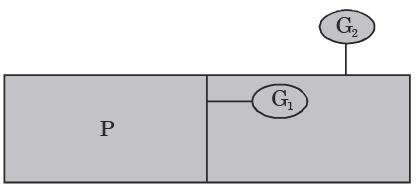Try yourself:A closed cylinder having a radius R and height H is filled with oil of density ρ. If the cylinder is rotated about its axis at an angular velocity of ω, the thrust at the bottom of the cylinder is



Try yourself:A cylindrical body of cross-sectional area A, height H and density ρs, is immersed to a depth h in a liquid of density ρ, and tied to the bottom with a string. The tension in the string is ____.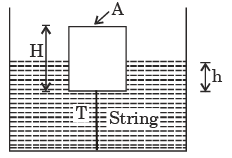Try yourself:A static fluid can have



Try yourself:The horizontal and vertical hydrostatic forces Fx and Fy on the semicircular gate, having a width w into the plane of figure, are



Try yourself:In given figure, if the pressure of gas in bulb A is 50 cm Hg vacuum and patm = 76 cm Hg, then height of column H is equal to



Try yourself:Refer to figure, the absolute pressure of gas A in the bulb is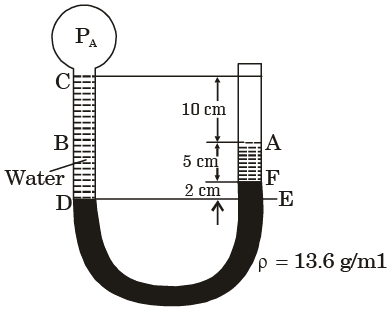Try yourself:A mercury manometer is used to measure the static pressure at a point in a water pipe as shown in figure. The level difference of mercury in the two limbs is 10 mm. The gauge pressure at that point is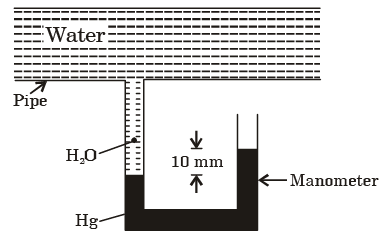Try yourself:The force F needed to support the liquid of density ρ and the vessel on top (fig.) is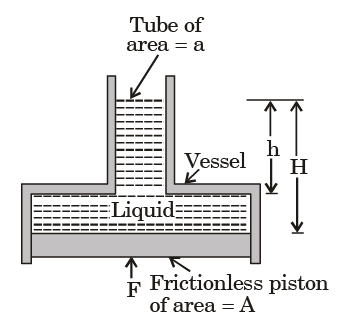Try yourself:The cross-sectional area of one limb of a U-tube manometer (figure shown below) is made 500 times larger than the other, so that the pressure difference between the two limbs can be determined by measuring h on one limb of the manometer. The percentage error involved is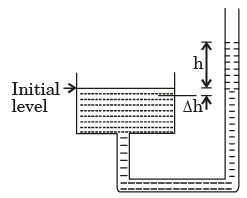Try yourself:A circular plate 1 m in diameter is submerged vertically in water such its upper edge is 8 m below the free surface of water. The total hydrostatic pressure force on one side of plate is



The document GATE Past Year Questions: Fluid Statics Notes | Study Fluid Mechanics - Mechanical Engineering is a part of the Mechanical Engineering Course Fluid Mechanics.
All you need of Mechanical Engineering at this link: Mechanical EngineeringUse Code STAYHOME200 and get INR 200 additional OFF

## Fluid Mechanics

47 videos|84 docs|73 tests

Track your progress, build streaks, highlight & save important lessons and more!

,

,

,

,

,

,

,

,

,

,

,

,

,

,

,

,

,

,

,

,

,

;